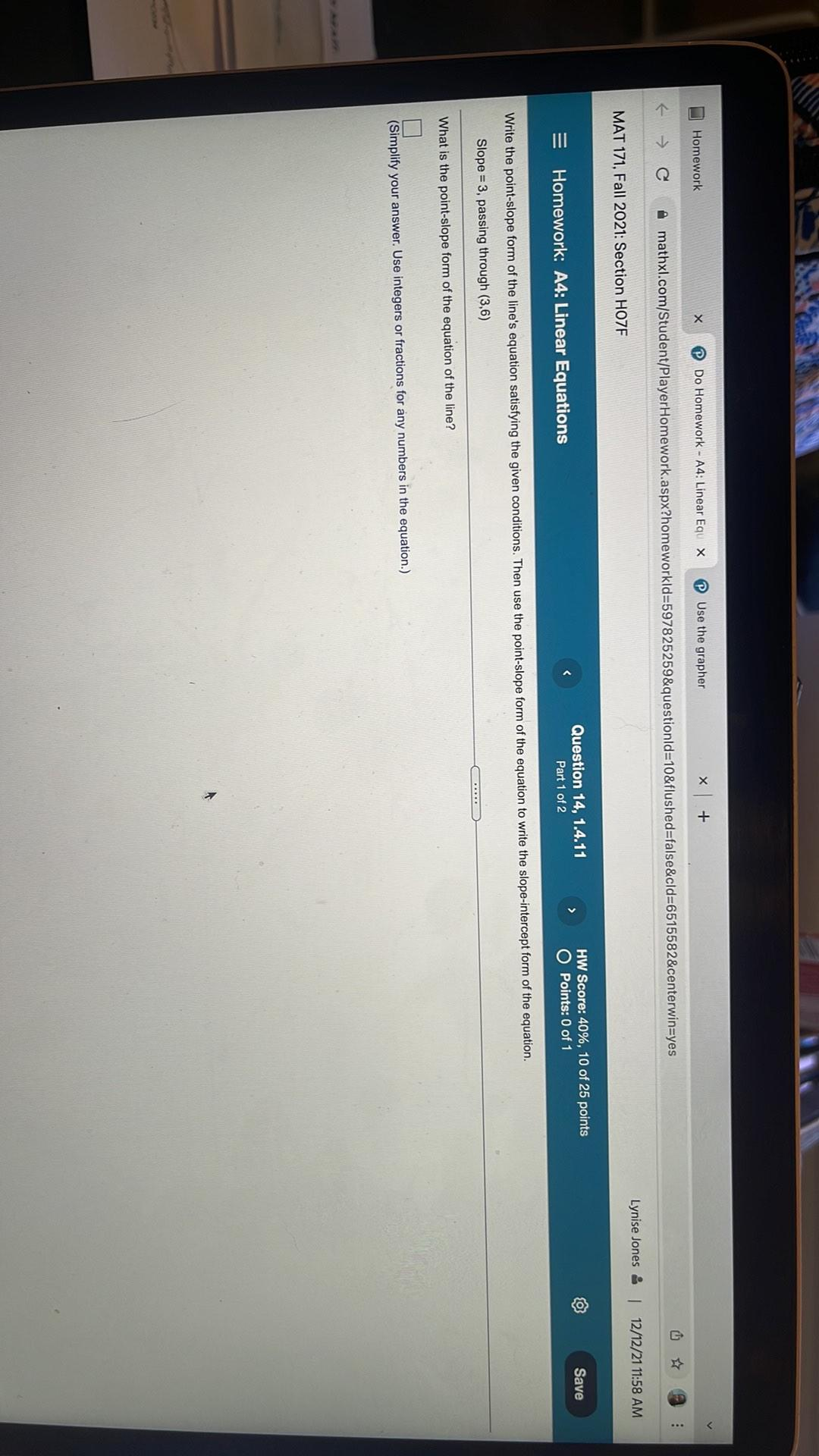Question:

# x Homework X Use the grapher Do Homework - A4: Linear Equ x + C : c mathxl.com/Student/Player Homework.aspx?homeworkld=597825259x Homework X Use the grapher Do Homework - A4: Linear Equ x + C : c mathxl.com/Student/Player Homework.aspx?homeworkld=597825259&questionId=10&flushed=false&cld=6515582&centerwin=yes Lynise Jones & 12/12/21 11:58 AM MAT 171, Fall 2021: Section HOZF HW Score: 40%, 10 of 25 points O Points: 0 of 1 Save Question 14, 1.4.11 Part 1 of 2 = Homework: A4: Linear Equations Write the point-slope form of the line's equation satisfying the given conditions. Then use the point-slope form of the equation to write the slope-intercept form of the equation. Slope = 3, passing through (3,6) What is the point-slope form of the equation of the line? (Simplify your answer. Use integers or fractions for any numbers in the equation.)